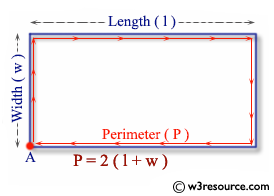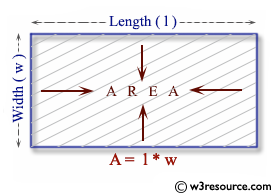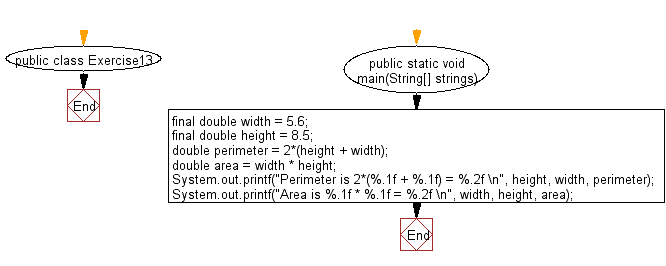﻿ Java exercises: Print the area and perimeter of a rectangle - w3resource# Java Exercises: Print the area and perimeter of a rectangle

## Java Basic: Exercise-13 with Solution

Write a Java program to print the area and perimeter of a rectangle.

Java: Perimeter of a rectangle

A perimeter is a path that surrounds a two-dimensional shape. The word comes from the Greek peri (around) and meter (measure). The perimeter can be used to calculate the length of fence required to surround a yard or garden. Following image represents the perimeter of a rectangle.Java: Area of a rectangle

In Euclidean plane geometry, a rectangle is a quadrilateral with four right angles. To find the area of a rectangle, multiply the length by the width.
A rectangle with four sides of equal length is a square.
Following image represents the area of a rectangle.

Pictorial Presentation:Sample Solution:

Java Code:

``````public class Exercise13 {

public static void main(String[] strings) {

final double width = 5.6;
final double height = 8.5;

double perimeter = 2*(height + width);

double area = width * height;

System.out.printf("Perimeter is 2*(%.1f + %.1f) = %.2f \n", height, width, perimeter);

System.out.printf("Area is %.1f * %.1f = %.2f \n", width, height, area);
}
}
```
```

Sample Output:

```Perimeter is 2*(8.5 + 5.6) = 28.20
Area is 5.6 * 8.5 = 47.60
```

Flowchart:Java Code Editor:

What is the difficulty level of this exercise?

Test your Programming skills with w3resource's quiz.

﻿

## Java: Tips of the Day

How to convert String to long in Java?

Use Long.parseLong()

``` Long.parseLong("0", 10)        // returns 0L
Long.parseLong("473", 10)      // returns 473L
Long.parseLong("-0", 10)       // returns 0L
Long.parseLong("-FF", 16)      // returns -255L
Long.parseLong("1100110", 2)   // returns 102L
Long.parseLong("99", 8)        // throws a NumberFormatException
Long.parseLong("Hazelnut", 10) // throws a NumberFormatException
Long.parseLong("Hazelnut", 36) // returns 1356099454469L
Long.parseLong("999")          // returns 999L
```

Ref: https://bit.ly/3cG9e16